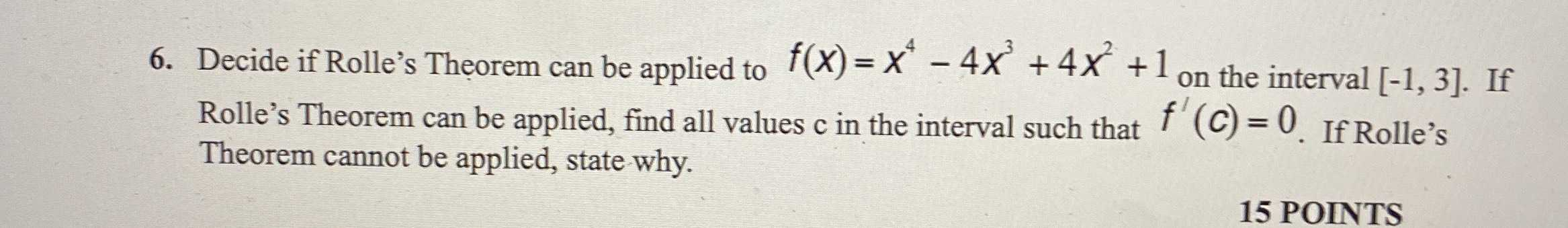### Still have math questions?

Algebra
QuestionDecide if Rolle's Theorem can be applied to $$f ( x ) = x ^ { 4 } - 4 x ^ { 3 } + 4 x ^ { 2 } + 1$$ on the interval $$[ - 1,3 ]$$ . If Rolle's Theorem can be applied, find all values $$c$$ in the interval such that $$f ^ { \prime } ( c ) = 0$$ . If Rolle's Theorem cannot be applied, state why.

Your input: find all numbers $$c$$ (with steps shown) to satisfy the conclusions of the Mean Value Theorem for the function $$f = x ^ { 4 } - 4 x ^ { 3 } + 4 x ^ { 2 } + 1$$ on the interval $$[ - 1,3 ]$$ .

The Mean Value Theorem states that for a continuous and differentiable function $$f ( x )$$ on the interval $$[ a , b ]$$ there exists such number $$c$$ from the interval $$( a , b )$$ , that $$f ^ { \prime } ( c ) = \frac { f ( b ) - f ( a ) } { b - a }$$

First, evaluate the function at the endpoints of the interval:

$$f ( 3 ) = 10$$

$$f ( - 1 ) = 10$$

Next, find the derivative: $$f ^ { \prime } ( c ) = 4 c ^ { 3 } - 12 c ^ { 2 } + 8 c$$

Form the equation: $$4 c ^ { 3 } - 12 c ^ { 2 } + 8 c = \frac { ( 10 ) - ( 10 ) } { ( 3 ) - ( - 1 ) }$$

Simplify: $$4 c ^ { 3 } - 12 c ^ { 2 } + 8 c = 0$$

Solve the equation on the given interval: $$c = 0 , c = 1 , c = 2$$

Answer: $$0,1,2$$

Solution
View full explanation on CameraMath App.